# Table Chart based Questions Notes | Study Logical Reasoning (LR) and Data Interpretation (DI) - CAT

## CAT: Table Chart based Questions Notes | Study Logical Reasoning (LR) and Data Interpretation (DI) - CAT

The document Table Chart based Questions Notes | Study Logical Reasoning (LR) and Data Interpretation (DI) - CAT is a part of the CAT Course Logical Reasoning (LR) and Data Interpretation (DI).
All you need of CAT at this link: CAT

Table Chart is the simplest form of presenting the data and most widely used in Data Interpretation section of CAT examination.
• The boxes of the table consist of different types of information like facts and figures, etc.
• Generally, the first row and column of the table denote the titles. Unlike other DI questions such as Pie Charts or Bar Graphs, it is more difficult and time-consuming to interpret data in tabular form.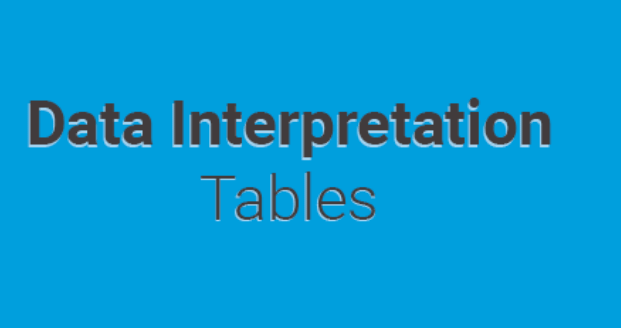Let's have a look at some solved questions based on Table Chart:

Q.1. The following table gives the percentage distribution of the population of five states, P, Q, R, S, and T, on the basis of the poverty line and also on the basis of sex.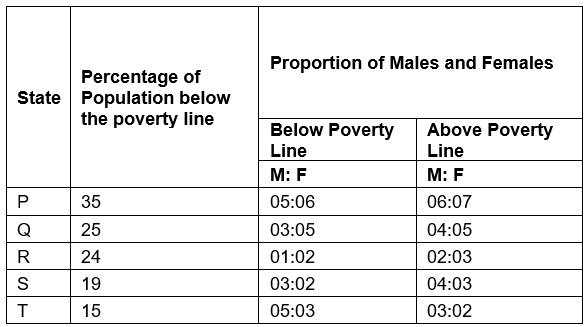Try yourself:What will be the male population above poverty line for State P if the female population below poverty line for State P is 2.1 million?

(i) If the male population above the poverty line for State R is 1.9 million, then the total population of State R is?

(a) 4.5 million
(b) 4.85 million
(c) 5.35 million
(d) 6.25 million

Ans. Option (d)

Explanation: Let the total population of State R be x million.

Then, the population of State R above the poverty line: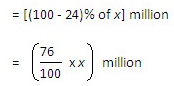And so, the male population of State R above the poverty line: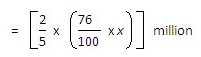But, It is given that the male population of State R above poverty line = 1.9 million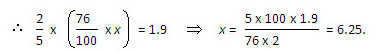∴ The total population of State R = 6.25 million

(ii) What will be the number of females above the poverty line in State S if it is known that the population of State S is 7 million?
(a) 3 million
(b) 2.43 million
(c) 1.33 million
(d) 5.7 million

Ans. Option (b)

Explanation: Total population of State S = 7 million.
∴ Population above poverty line = {100 - 19}% of 71 million = 81% of 7 million = 5.67 million.
And so, the number of females above the poverty line in State S: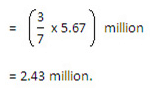(iii) If the population of males below the poverty line for State Q is 2.4 million and that for State T is 6 million, then the total populations of States Q and T are in the ratio?

(a) 1:3
(b) 2:5
(c) 3:7
(d) 4:9

Ans. Option (b)

Q.2. Study the following table and answer the questions based on it.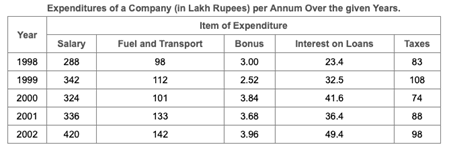Try yourself:What is the average amount of interest per year which the company had to pay during this period?

(i) Total expenditure on all these items in 1998 was approximately what percent of the total expenditure in 2002?

(a) 62%

(b) 66%

(c) 69%

(d) 71%

Ans. Option (c)

Explanation: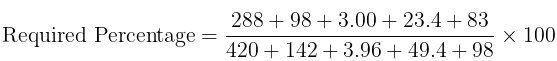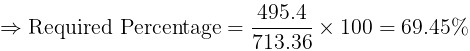Try yourself:The total expenditure of the company over these items during the year 2000 is?

(ii) The ratio between the total expenditure on Taxes for all the years and the total expenditure on Fuel and Transport for all the years respectively is approximate?

(a) 4:7

(b) 10:13

(c) 15:18

(d) 5:8

Ans. Option (b)

Explanation: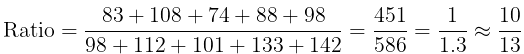Try yourself:The total amount of bonus paid by the company during the given period is approximately what percent of the total amount of salary paid during this period?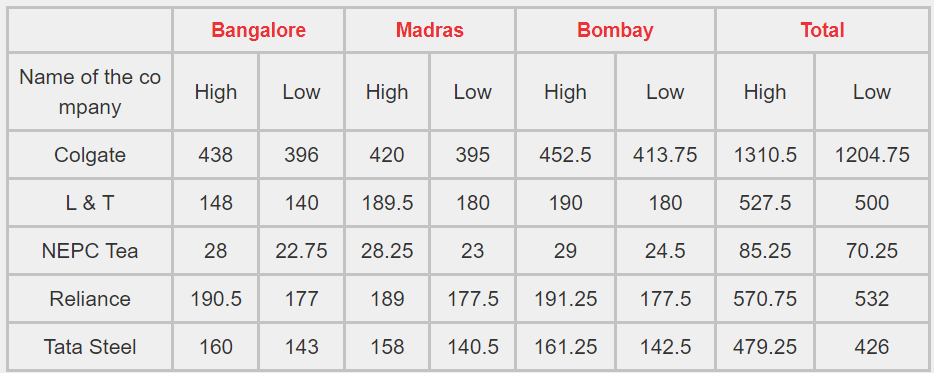(i) The average of the high rates of share in all the three Stock Exchanges for NEPC Tea is

1. Rs.28.422.
2. Rs.27.423.
3. Rs.29.14.
4. Rs.28.93

Solution: The last column is the total of all three stock exchanges (high rates and low rates, resp.) for every company. So, the average for NEPC high rates = 85.25/3= Rs. 28.42. Hence, the answer is 1st option.

Alternatively,

We know that, average has to lie somewhere in between the two extreme values. Here, the values are 28, 28.25 and 29. The second and third options are out of this range and fourth option is too close to the higher end. Thus, the only option left is the first one and hence, that is the answer.

(ii) For Reliance, the low rate of share is less than the average of the low rates of shares of the same company, in the Stock Exchange at

2. Bombay

3. Bangalore

4. None of these

Solution: Average of low rates of shares of Reliance = (177.5+177.5+177)/3> 177, which is the low rate of share of Reliance at Bangalore stock exchange. So, the correct option is 3.

Alternatively,

We know that, the city having the smallest value will be lesser than the average and the city having the largest value will be definitely more than the average. Using this logic, the answer can be marked as Bangalore.

(iii) For Tata Steel, the ratio of the high rate of share to the low rate is maximum in the Stock Exchange at

1. Bangalore

3. Bombay

4. None of these

Solution: At Bangalore Stock Exchange, ratio of high rate to low rate = (160/143) = 1.11;

At Madras Stock Exchange, ratio of high rate to low rate = (158/140.5) = 1.12;

At Bombay Stock Exchange, ratio of high rate to low rate = (161.25/142.5) = 1.13.

As the maximum ratio is in Bombay, it will be the answer. Hence, the answer option is 3.

(iv) The low rate of share in L & T at Madras Stock Exchange bears a ratio, to the total low rate of share of the same company, of

1. 8/19

2. 9/20

3. 11/25

4. 9/25

Solution: Low rate of share of L & T at Madras Stock Exchange = Rs. 180.

Total of low rate of share of L & T in all three Stock Exchanges = Rs. 500.

Ratio of (i)/(ii)= 180/500 = 9/25. Hence, the answer is 4th option.

Q.4. Study the following table and answer the questions based on it.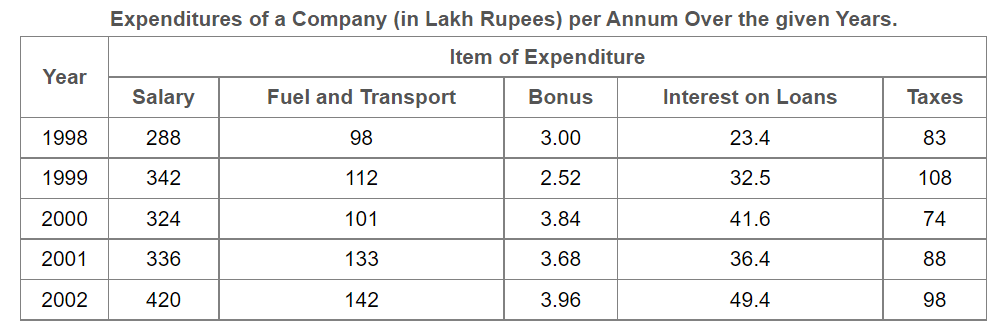(i)  What is the average amount of interest per year which the company had to pay during this period?

1. Rs. 32.43 lakhs
2. Rs. 33.72 lakhs
3. Rs. 34.18 lakhs
4. Rs. 36.66 lakhs

Explanation: Average amount of interest paid by the Company during the given period.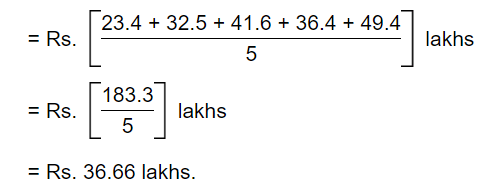(ii) The total amount of bonus paid by the company during the given period is approximately what percent of the total amount of salary paid during this period?

1.    0.1%

2.    0.5%

3.    1%

4.    1.25%

Explanation: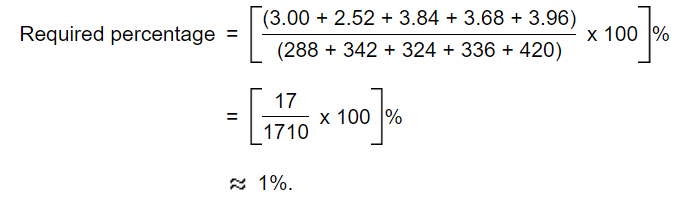(iii) Total expenditure on all these items in 1998 was approximately what percent of the total expenditure in 2002?

1.    62%
2.    66%
3.    69%
4.     71%

Explanation: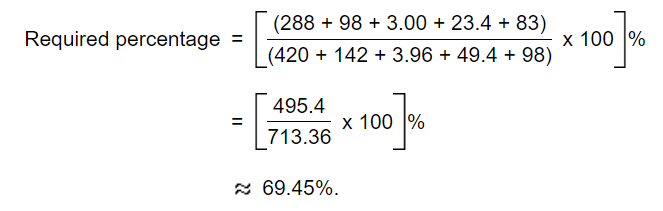The document Table Chart based Questions Notes | Study Logical Reasoning (LR) and Data Interpretation (DI) - CAT is a part of the CAT Course Logical Reasoning (LR) and Data Interpretation (DI).
All you need of CAT at this link: CATUse Code STAYHOME200 and get INR 200 additional OFF

## Logical Reasoning (LR) and Data Interpretation (DI)

55 videos|53 docs|102 tests

### How to Prepare for CAT

Read our guide to prepare for CAT which is created by Toppers & the best Teachers

Track your progress, build streaks, highlight & save important lessons and more!

,

,

,

,

,

,

,

,

,

,

,

,

,

,

,

,

,

,

,

,

,

;